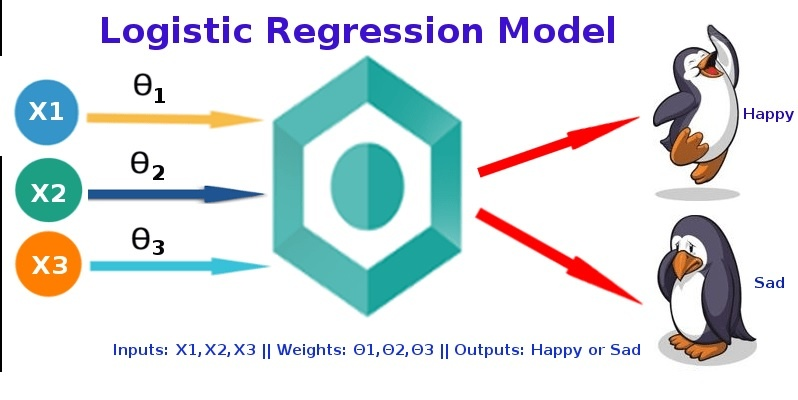# Logistic Regression – The major Algorithm for Data Science

A lot of things have changed over a few decades. From the way people communicated, traveled and ultimately, lived. Today, everything is easier and way more accessible than it was before. One very obvious reason is the advancement in technology or to be more precise, the introduction of  Artificial Intelligence, Data Science, Cloud Storage, and Machine Learning.

Data science is a very vast subject and one of the hottest topics in recent times. From data mining, speech recognition to all other techs that have resulted in the advancement are all result of Data Science. Not only that, over the past 3 years, one job that has seen rapid growth is that of a Data Scientist. This job has witnessed a rise of over 75% in the majority of the developed countries.These facts are enough to prove that Data Science is here to stay and rule. Now, that we know it’s importance and need, let’s focus on one of it’s the most important algorithm, the Logistic Regression.

### What is Logistic Regression?

Introduced more than half a century ago, in 1958, by a British Statistician David Cox. Logistic Regression is the most suitable regression analysis when the dependent variable (target) is categorical. It is a predictive analysis just like other regression analyses. Logistic regression is used to describe the relationship between a dependent binary variable and one or more nominal, ordinal, interval or ratio-level independent variables.

### Logistic Regression has several

Uses in modern times as well as when it was introduced. Back then, in the early twentieth century, it was used in the biological sciences. Well, that wasn’t the only use. Many scholars used logistic regression to describe data in Social Science applications.### Use of Logistic Regression at Present Time

Today, as we have advanced in science, so has the usage of Logistic Regression. The most common use is seen in Medical science in terms of Trauma and Injury Severity Score or TRISS. It calculates the survival chances or the mortality rate of a patient. Other common uses include prediction of risk in determining how much a patient is likely to develop a certain disease based on his observed characteristics. Logistic Regression is highly applicable in predicting the votes based on a voter’s attributes and past experience.

Its reach is not just limited to biological science and social science, but the new age science of ‘Machine learning’ finds logistic regression pretty useful too. In fact, after linear regression, Logistic regression is the most prominent machine learning algorithm. Unlike the linear regression which predicts or forecasts, the logistic regression is more absolute as it helps in classification.

Logistic Regression is a part of data science, yet it’s one of its major algorithms. Precisely, due to its multi-functionality in various fields.